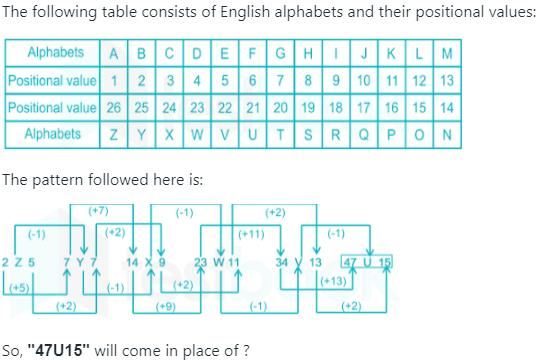Courses

# Olympiad Test : Series And Pattern - 1

## 20 Questions MCQ Test Math Olympiad for Class 5 | Olympiad Test : Series And Pattern - 1

Description
This mock test of Olympiad Test : Series And Pattern - 1 for Class 5 helps you for every Class 5 entrance exam. This contains 20 Multiple Choice Questions for Class 5 Olympiad Test : Series And Pattern - 1 (mcq) to study with solutions a complete question bank. The solved questions answers in this Olympiad Test : Series And Pattern - 1 quiz give you a good mix of easy questions and tough questions. Class 5 students definitely take this Olympiad Test : Series And Pattern - 1 exercise for a better result in the exam. You can find other Olympiad Test : Series And Pattern - 1 extra questions, long questions & short questions for Class 5 on EduRev as well by searching above.
QUESTION: 1

### Complete the series ​____ op ____ mo ____n------pnmop____

Solution:

The pattern ‘mopn’ is repeated.

m o p n | m o p n | m o p n | m o p n
Thus following the above series, the missing terms will be: mnpmon

QUESTION: 2

### Complete the series ba____ba____bac____acb____cbac

Solution:

The series is bac/bac/bac/bac/bac/bac. The pattern bac is repeated.

QUESTION: 3

### Complete the series m____nm____n____ an____a____ ma____

Solution:

The series is man/man/man/man/man. The pattern ‘man’ is repeated.

QUESTION: 4

Complete the series
​R___MR____M__ ___M

Solution:

The series is RAM/RAM/RAM/RRD.
The pattern RAM is repeated.

QUESTION: 5

Complete the series
​bca____b____aabc ____a____caa

Solution:

The series is bcaa/bcaa/bcaa/bcaa. The pattern ‘bcaa’ is repeated.

QUESTION: 6

Complete the series
a____ba____b____b____a____b

Solution:

The series is abb/abb/abb/abb. The pattern ‘abb’ is repeated.

QUESTION: 7

Complete the series
___nmmn____mmnn____mnnm____

Solution:

The series is nnmm/nnmn/nnmm/ nnmm. The pattern ‘nnmm’ is repeated.

QUESTION: 8

Complete the series
____bc-------b ____aabc

Solution:

The series is abc/cab/bca/abc. The letters are in cyclic order.

QUESTION: 9

Complete the series
cccbb____aa____cc____bbbaa____c

Solution:

The series is ccc bbb aaa/ccc bbb aaa/c. The pattern ccc bbb aaa is repeated.

QUESTION: 10

Complete the series

Solution:

QUESTION: 11

Find the next two elements in series:
A/2, B/4, C/6, D/8

Solution:

The letters are in alphabetical order. The numbers increase by two.

QUESTION: 12

Find the missing element: Q1F, S2E, U6D, W21C, ?

Solution:

The first letter moves two steps forward. The last letter moves one step backward. The number series runs as below :
1 x 1 + 1 = 2
2 x 2 + 2 = 6
6 x 3 + 3 = 21
21 x 4 + 4 = 88

QUESTION: 13

Find the missing element: 2Z5, 7Y7, 14X9, 23W11, 34V13, ?

Solution:QUESTION: 14

Find the missing element: P3C, R5F, T8I, V12L, ?

Solution:

The first letter of the terms are alternate. The sequence followed by the numbers is +2, +3, +4,.... The last letter is three steps ahead of the last letter of the preceding term.

QUESTION: 15

Find the missing element:

J2Z, K4X, 17V, ?, H16R, M22P

Solution:

This pattern consists combination of two series for first place and other two terms vary with two different series as :

For first place : J,I, H, G, ...... and K, L, M, N, .....

For second place : 2, 4, 7, 11, 16, 22, ......

and for third place : Z, X, V, T, R,P, ......

∴Missing Term=L11T.

QUESTION: 16

Find the missing element: 3F, 6G, 11I, 18L, ?

Solution:

The letters move 1, 2, 3, ... steps forward. The numbers increase by + 3, + 5, + 7, + 9....

QUESTION: 17

Find the missing element: D-4, F-6, H-8, J-10, ?, ?

Solution:

The letters are alternate and the numbers indicate their position in the alphabet from the beginning.

QUESTION: 18

Find the missing element: D-4, F-6, H-8, J-10, ?, ?

Solution:

The letters are alternate and the numbers indicate their position in the alphabet from the beginning.

QUESTION: 19

Find the missing element:
2A11, 4D13, 12G17, ?

Solution:

The first numbers in the terms follow the sequence x 2, x 3, x 4. The letter moves three steps forward. The last numbers follow the sequence + 2, + 4, + 6.

QUESTION: 20

Find the missing element: C4X, F9U, I16R, ?

Solution:

1st letter : C + 3 = F + 3 = I + 3 = L
Number : 4 (22 ) → 9 (32 ) → 16(42 ) → 25 (52 )
3rd letter : X - 3 = U - 3 = R - 3 = O